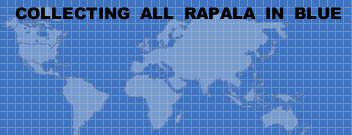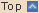The GOLD BELLY PERCH is a very old color pattern. This color was introduced in 1977. It was made only for a short while in the 1970s and succeeded by the foil pattern as known today. <<<<<<<<<<<<<<<<<<<<<<<<<<<<<<<<<<<<<<<<<<<<<<<<<<<<<<<<<<<<<<<<<<<<
A full set of Floating Original GBP
(plus MFR-3 GBP)Seite 1 von 1fischerl
cdj-7-11@hotmail.de